# Finding current through a resistor

freshcoast
1. Problem statement.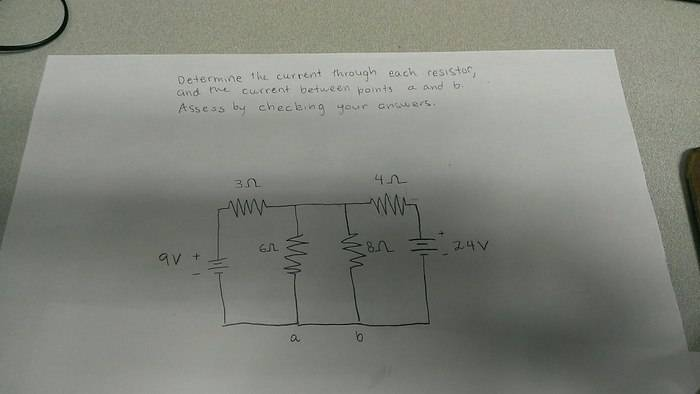2. Known equations
Kirchoffs law, logic

3. Attempt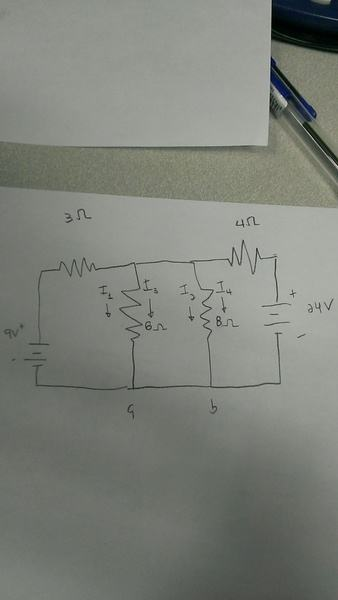I believe there are 4 currents, as shown above. i1 and i2 are coming from the 9 volt battery in a clockwise direction and i3 and i4 are from the 24v battery in a anti clockwise direction. I just apply kirchkoffs law for each current with the resistor in its path way.

Ex. For i1 the equation would look like

9v - i1(3ohms) -i1(6ohms) = 0

Then

A = i1 + i3
B = i2 + i4

## Answers and Replies

Mentor
freshcoast
Hmm, what I learned is at junctions, current in = current out. Would that mean that there will only be 2 currents? For example from the 9 volt battery, the current would travel clockwise through the 3ohm resistor and it has only one of two choices to go after that, which would be down to the 6 ohm resistor or towards the 4 ohm resistor?

Staff Emeritus
Hmm, what I learned is at junctions, current in = current out.
Yes, let's use that.

Would that mean that there will only be 2 currents? For example from the 9 volt battery, the current would travel clockwise through the 3ohm resistor and it has only one of two choices to go after that, which would be down to the 6 ohm resistor or towards the 4 ohm resistor?
No, it has 3 choices, there are 3 paths to get back to the - terminal of its 9V source, one path you have overlooked being through the 24V source.

The easiest (?) way to analyze this is to let the voltage across the 6Ω resistor be Vx volts.Now, express the current through every resistor (and voltage source) in terms of that one unknown, Vx.

See how you go.

Eventually, you'll be able to solve for that unknown, and once you have, you'll know the current in every element.

freshcoast
I am having trouble setting up the equation still. I am thinking there might be 2 equations max, where the current is coming from the 9v battery and the 24volt battery. I am also wondering if I should treat the currents as the same value as for each of the resistors.

For example, this is what i'm thinking the equations would look like.

9v - i1(3ohm) - i1(6ohm) - i1(8ohm) - i1(4ohm) - 24v - Vx = 0

And then do the same again for the other voltage source.

Mentor
If you use NascentOxygen's suggestion and set the potential at the top of the middle resistors to Vx (the potential is with respect to a common reference point assumed to be the bottom node of the circuit), then you can write expressions for every current using Vx and the other known potentials. For example, the current from the Vx node to the 9V battery through the 3 Ω resistor would be

##I = \frac{Vx - 9}{3}##

If you wrote similar expressions for all the currents for the Vx node and summed them (i.e. doing KCL at the Vx node) and set the result to zero, you'd have one equation in one unknown (Vx). Solve for Vx. Then knowing Vx you can go back and give values to all those individual currents.

The above procedure constitutes "Nodal Analysis", which you will cover in your course at some point. But the technique should be pretty obvious if you already know KCL and KCL.

freshcoast
I see, so if I put a node at the top of the middle resistors, (between the 3ohm and 4ohm resistors) and do a similar equation to the current from 9v to that node, Would I get 2 other currents, which goes in a anti-clockwise circle from the 6 ohm and 8 ohm resistor, and one other current from the 24v battery through the 4 ohm resistor, and then I sum them all up and equal to zero, would that be my equation?

If so this is what I've got,

9(volt) - 3I -Vx = 0 (which is the example you provided, just haven't sold for I)
Vx - 6I - 8I = 0 (node, current going anti-clock wise through the 6 ohm and 8 ohm resistor)
24(volt) - 4I - Vx = 0

Then I add them all up,

33(volt) - 21I - Vx = 0

Vx = 33-21I

Does this seem correct?

Mentor
No. Don't unravel the expressions for individual currents. Leave them in the form I showed. The "I" in the expression I wrote was not meant to be taken as a new variable, it's only meant to show that the expression yields a current value; it's the current in that particular branch. I suppose I should have taken your circuit diagram and placed new current labels on everything. So here: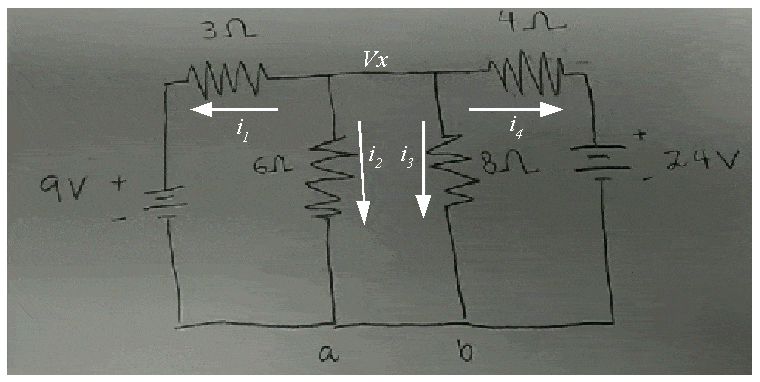The "I" in the expression I wrote corresponds to ##i_1## in the above diagram.

This is KCL you're doing: The sum of all currents entering or leaving a single node is zero. The middle node has 4 branches leading from it so there will be four expressions of the form that I showed, one for each branch leaving the node. Each expression is of the form:
$$\frac{\Delta V}{R}$$
The ##\Delta V## for the example I gave is Vx - 9, corresponding to the potential drop across the 3 Ω resistor that sits between Vx and the 9 V potential at the top of the 9 V battery. Hence the current in that branch is:
$$\frac{Vx - 9}{3}$$
That will be one term of four terms that go to make your KCL expression for the node:
$$\frac{Vx - 9}{3} + \frac{(~~~~)}{(~)} + \frac{(~~~~)}{(~)} + \frac{(~~~~)}{(~)} = 0$$
which corresponds to the KCL statement: ##i_1 + i_2 + i_3 + i_4 = 0## for the diagram above; each term represents one of the currents.

Once you solve for Vx you can revisit the individual terms to find the individual currents.

#### Attachments

freshcoast
Hmm, well using your examples, I've come up with the currents as following,

i1 = Vx-9 / 3

i2 = Vx-9 / 9 ,I am picturing this current came from the 9v battery and went through the 3 ohm resistor then down to the 6 ohm resistor

i3 = Vx-24 / 12 , this one same like above but the opposite side, from the 24v battery through the 4 ohm resistor and then down the 8 ohm resistor.

i4 = Vx-24 / 4 , this one I figured by using the same logic as the example you've given me

I am also confused, the equation I was taught was

V - IR = 0 (v for voltage source)

but the way you have i1 set up it looks like it is

V + IR = 0

Is that a typo or is it a different equation we are using here?

Staff Emeritus
i2 = Vx-9 / 9
That wire along the bottom is 0 volts. It links up the 0V end of one battery and the 0V end of the other battery, and it's zero volts all the way along it.

Where the top of a battery is +9V, the bottom is 0V.

So, using Ohms Law: i2 = (Vx - 0) / 6

(That - sign on a cell is a bit misleading. The bottom of the battery is not -9V, it's 0V.)

Last edited:
freshcoast
Am I picturing the node in the middle as a 0v battery? so shouldn't the i2 current, from the node, go down the 6 ohm resistor, then back up to the 8 ohm resistor?

so

i2 = Vx / 14 ?

freshcoast
hmm, so i3 would then be

i3 = (Vx - 0 ) / 8

I am having trouble picturing how the current flows or the possibilities that the current could flow, is there any tips or ways to make that more clear?

from what I've read from the equations I am picturing this is what is happening, which I know is incorrect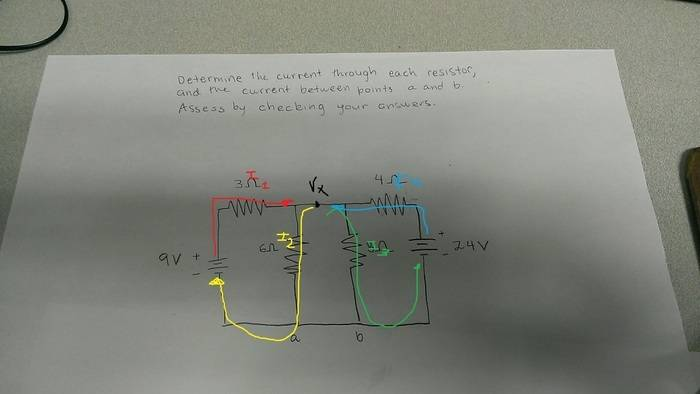Staff Emeritus
Even were you right, in forming these equations we aren't interested in any current's full path.

We are applying Ohms Law to individual branchs (resistors). The 6 Ohm resistor has Vx volts across it, and its current is I2. That's all you need to know to form the equation.

As to your question, current goes from a higher potential to a lower potential. So if it goes from +Vx volts down to 0V, it's not going to then reverse and go from 0V back up to +Vx volts through another resistor. After reaching the ground wire (0V) current returns to the battery from whence it originated (and it's pumped back up to that battery's positive potential).

EDIT: the diagram you have just added, with coloured paths, is close enough.

freshcoast
I see, just to make sure, would the current between points a and b be i2 = a , and i3 = b or is it 0 since its a ground wire?

Staff Emeritus
I see, just to make sure, would the current between points a and b be i2 = a , and i3 = b or is it 0 since its a ground wire?
That doesn't make any sense.Point a is a dot marked on a schematic. I2 is a current and it has units of amperes.

Strictly speaking, points a and b are one and the same, they are that bottom node, and the voltage all along it is zero volts.

But I can see what you mean, regarding that as a sketch of the physical layout. If you were to add an ammeter in between points a and b, what value of current would it measure? It would measure exactly the difference between the current from the battery and the current through the 6Ω, because at point a the current through the 6Ω joins with the current between nodes b and a to form the current into the base of the 6V battery.

Mentor
freshcoast, here's your circuit redrawn with points of interest indicated. The bottom rail (blue) is all one rail and is a convenient place to put our reference point for all potentials in the circuit. Hence the 0 V designation and the ground symbol on the bottom left.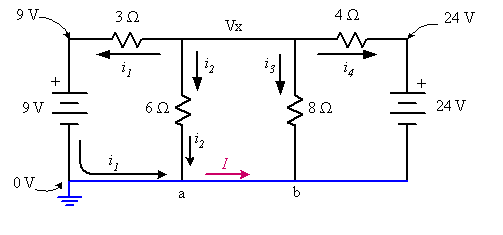The nodes at the top left and top right have their potentials fixed at +9 V and +24 V by the batteries (the batteries are directly between those points and the reference node).

Now, you're looking to find the current between points a and b assuming that in a real implementation of this circuit there would exist a wire corresponding to a-b. I've designated that current as I in the diagram. Consider point a as a node. How might you determine what I is?

#### Attachments

freshcoast
Sum of current in = sum current out?

So with your diagram, I1 + I2 = I?

Mentor
Sum of current in = sum current out?

So with your diagram, I1 + I2 = I?

That's the idea. Now all you need to do is solve for I1 and I2. Hence the nodal analysis...

freshcoast
I am confused about how to check my answers, I know its correct but I don't know why, for the currents my values are

I1 = 0.43A
I2 = 1.71A
I3 = 1.285A
I4 = -3.43A

I know that to make sure I'm correct all the voltages need to add up properly, but I do not see the reasoning behind this. For example, if I do

V1 = I1 * R

Which is

V1 = (0.43A) * ( 3ohm) = 1.29V,

I'm thinking 1.29 volts get dropped after passing the 3 ohm resistor? But why is I2 which I calculated as 10.26v, which is higher than the 9v battery? I don't know I'm just having trouble grasping the general concept of how the voltages should add up and where.

Thanks so far for all the input I've received

freshcoast
Oh and also for future problems,

Is the best way to solve these is to look for a symmetrical location on the circuit, label that as a node with voltage Vx, then just write out current equations per each of the one resistor? So no current equation should have no more than one resistor value on it?

Mentor
I am confused about how to check my answers, I know its correct but I don't know why, for the currents my values are

I1 = 0.43A
I2 = 1.71A
I3 = 1.285A
I4 = -3.43A

I know that to make sure I'm correct all the voltages need to add up properly, but I do not see the reasoning behind this. For example, if I do

V1 = I1 * R

Which is

V1 = (0.43A) * ( 3ohm) = 1.29V,

I'm thinking 1.29 volts get dropped after passing the 3 ohm resistor? But why is I2 which I calculated as 10.26v, which is higher than the 9v battery? I don't know I'm just having trouble grasping the general concept of how the voltages should add up and where.

Thanks so far for all the input I've received

If all the currents are correct then KCL will work for each node and KVL will work for any closed path ("circuit"). The drop across the 6 Ω resistor can be higher than the battery on the left because the battery on the right is also supplying its current, and that battery is 24 V. So 10.26 V is perfectly reasonable for an individual voltage drop in this circuit.

Mentor
Oh and also for future problems,

Is the best way to solve these is to look for a symmetrical location on the circuit, label that as a node with voltage Vx, then just write out current equations per each of the one resistor? So no current equation should have no more than one resistor value on it?

Really it depends upon the individual circuit and your skill at recognizing opportunities for simplifying the analysis.

There are a handful of techniques that come in handy and while all of them will work, some may be easier to use for a given situation and circuit than others. Symmetry plays a role in recognizing such opportunities, but what really counts is experience gained through practice.

Nodal analysis is a powerful method when you need to know the voltages in a circuit. Here it was applied to find currents because it let you find a very important voltage (Vx) that held the key to finding all the currents easily, and Vx could be found with a single equation. That's economy!

Mesh analysis is another technique you'll come across, and it's very handy for finding currents in circuits. It's like KVL loop equations but with a twist that makes them particularly easy to write by inspection.

There are several more techniques that I won't bother listing here, but they'll be introduced in your courses as they become useful for the material.

Staff Emeritus
I am confused about how to check my answers, I
It's handy to look for a second way to solve the problem. Try this:

(i) Replace the 24V battery by a simple wire (a short circuit), leaving you with a circuit comprising the 9V battery and 4 resistors. Determine the current in the 6Ω under these conditions. (Can you see 3 resistors in parallel?)

(ii) Replace the 9V battery by a wire. With the circuit now comprising a 24V source and 4 resistors, determine the current in the 6Ω.

(iii) Sum the two currents and that's your answer for the total current in the 6Ω.Of course, this answer should tally with that using the unknown voltage Vx method.

This second method is based on 'superposition'.

freshcoast
Hmm, when I take out the 24v battery, I am seeing that the 3 ohm and 6 ohm resistor in a series in parallel with the 8 and 4 ohm resistor...and then I can find the R(eq) of that to find a current. But when I've found that current and try multiplying it to each resistor I am not getting the right amount of total voltage...

Staff Emeritus